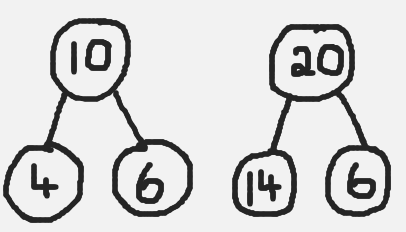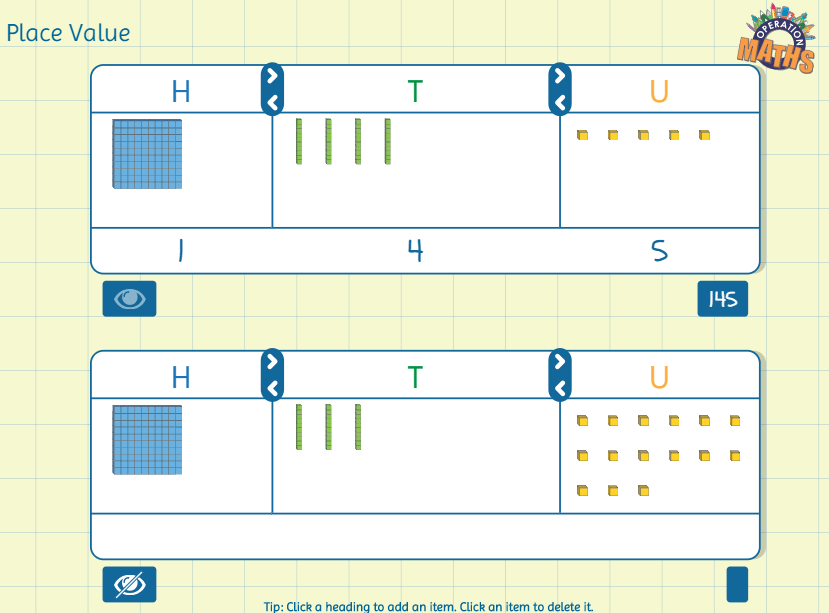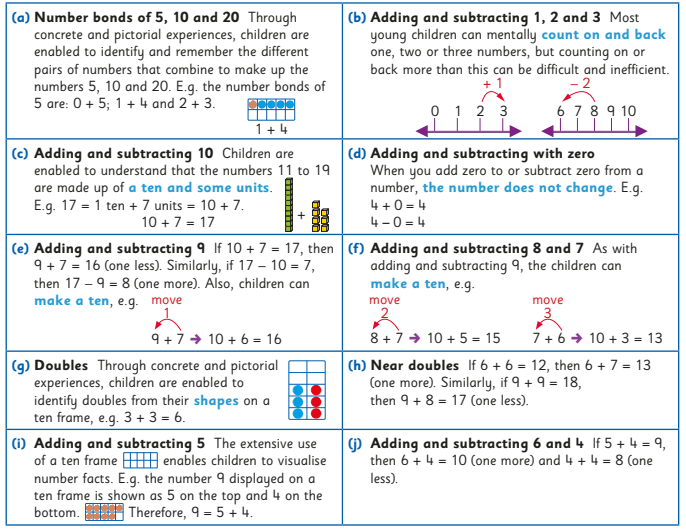# Digging Deeper into … Addition and Subtraction (infants to second class)

## Digging Deeper into … Addition and Subtraction (infants to second class)

Category : Uncategorized

A quick look at the maths curriculum for junior and senior infants will reveal that, within the strand of number, there are no strand units entitled operations, addition or subtraction, as are evident in the curriculum for first and second classes. However both operations are there – under the guise of combining, partitioning and comparing.

Addition and subtraction are two of the four basic mathematical operations (multiplication and division being the other two):

• Addition involves the joining/combining of two or more quantities/sets/parts to get one quantity/whole/set, typically referred to as the sum or total. There are two main types: active (2 children at a table and 3 more join them) or static (2 boys and 3 girls at a table, how many children in all?)
• There are three types of subtraction:
• take-away (active) which involves the removal/deduction of one quantity/part from a whole amount/quantity
• comparison (static) which involves identifying by how much one quantity/set is more or less than another (the difference)
• missing addend (active) which involves identifying the amount needed to combine with a known part to make a whole.

In each type of subtraction we know the total/whole and a part and we need to find the missing part, which could be the amount left, the difference or the missing addend.

The types of addition and subtraction are explained very clearly and succinctly in the Origo One videos below.

### Relationship between addition and subtraction

As shown in the videos above, addition and subtraction are inverse operations; we can demonstrate addition by adding more to an existing amount; the reverse action would involve removing an amount, thus demonstrating subtraction as take away. In contrast to traditional maths schemes, which often have separate chapters for each of these operations, Operation Maths predominantly teaches addition and subtraction together, as related concepts. Teaching the operations in this way will encourage the children to begin to recognise the relationships between addition and subtraction.

Beginning in first and second classes, the children are enabled to understand addition and subtraction as being the inverse of each other, which will progress towards using the inverse operation to check calculations in higher classes.

### CPA Approach within a context

As mentioned repeatedly in previous posts, both the Operation Maths and Number Facts series are based on a CPA approach. Furthermore, as was referenced in the videos above, for the children to develop a deep understanding of the different types of addition and subtraction, there has to be some context or story, with which they can identify. This, in turn, should be explored via progression through concrete, pictorial and abstract stages.

This context can be simply made up by the teacher or be inspired by a picture book that the class is reading. It can be modeled using the concrete materials available in the classroom (eg plastic animals, toy cars, play food etc. ) and/or using the Operation Maths Sorting eManipulative (see below) and the extensive suite of inbuilt images; the images can be shown either with or without a background (background options include five and ten frames, set outlines and various grids).HINT: To find out more about how to use the 5, 10 and 20 frames that accompany the Operation Maths series please read on here: http://operationmaths.ie/youve-been-framed-closer-look-ten-frames/

As the children progress, the need arises to record the operations using some graphic means. Initially, this can include representing each of the items in the story with counters and/or cubes. In turn, bar models could also be used to represent number relationships, while bearing in mind that different types of bar models will be required to model different context and types of subtraction (even though the subtraction sentences, if using them, might look exactly the same). Using the examples below, the first bar model (a part-whole bar model) could be used to model this story: Snow White had seven dwarfs. If four of these went to work, how many were left at home? Whereas, the second bar model (a comparison bar model) would better suit this story: the seven dwarfs all wanted to sit down at the table but there were only four chairs. How many dwarfs had no chair?While bar models do not specifically appear until in the pupils books until Operation Maths 3, the children could use and explore simple bar models. Thinking Blocks Jr is based on simple bar models and could be shown to the class on the IWB while the children suggest answers and labels on their Operation Maths MWBs.  Then the children could draw simple models in their books to help solve the word problems there. Furthermore , as shown above, the Bar Modelling eManipulative could also be used to create bars of different length.Before rushing too quickly into abstract recording (using only digits and symbols), an alternative intermediary stage could be to represent the relationships, using a branching bond (opposite). Similar to the part-whole bar model earlier, this branching bond structure encourages the children to appreciate that two sets/parts ( 4 and 6) can be combined to make a larger set/whole (10). Inversely, when a part (4) is removed from the whole (10), a part is left (6). This bond structure can also represent the missing addend type of subtraction: if a part was hidden (6), the question could be asked  what must be added to 4 to make 10.

Both branching bonds and simple bar models are used throughout the Number Facts series to represent relationships and demonstrate strategies. They are also used throughout the Operation Maths 3-6 books, but in increasingly more complex situations.

### The meaning of the equals sign

With the formal introduction of addition number sentences in senior infants (ie the recording of relationships using the plus and equals sign), followed by the formal introduction of subtraction sentences (using the minus sign) in first class, comes the need to correctly interpret the purpose of the equals sign as identifying equivalence; ie that the value on one side of the equals sign is the same as the value on the other side. It is essential at this stage that the children don’t interpret the equals signal incorrectly as being a signpost indicating that the answer is coming next. A pan or bucket balance is an extremely valuable resource to help demonstrate equivalency, as can be seen in the video below.

Calculations in the Operation Maths book are often shown vertically and horizontally. When presented horizontally, it is often misinterpreted that the children must now rewrite the calculation vertically, to be solved using the traditional column method (see more on the column method in the next section). Rather, presenting calculations horizontally is a deliberate effort to encourage the children to explore how to solve the calculation using a concrete based approach and/or using a mental strategy, as opposed to always tackling these calculations in a written way.

### Looking at more complex numbers

In first and second classes, once introduced to operations using two-digit numbers, children can often have tunnel vision (or column vision) regarding addition and subtraction calculations: they “do” the units, and then the tens, without really looking at the whole numbers or the processes involved.

One way in which you can encourage the children to look at and understand these operations better is by using a CPA approach. This means that the children’s initial experiences should involve groupable base ten concrete materials (e.g. bundling straws or lollipop sticks, ten-frames and counters, unifix or multi-link cubes arranges in sticks of ten, see below), where a ten can be physically decomposed  into ten units and vice versa, before moving on to pregrouped base ten materials (eg base ten blocks/Dienes blocks, base ten money and/or Operation Maths place value discs) which require a swap to exchange a ten for ten units and vice versa.When children are comfortable with the manipulating the concrete materials, they can move on to a process whereby these materials are represented pictorially and/or demonstrate the process using a suitable the visual structure eg an empty number line and/or bar model. Abstract exercises, where the focus is primarily on numbers and/or digits, should only appear as part of the final stage of this process.When exchanging tens and units (or tens and hundreds in second class), reinforce that a ten is also the same as 10 units, and that a hundred is the same as 10 tens and is the same as 100 units. The use of non-canonical arrangements of numbers (e.g. representing 145 as 1H 3T 15U or  14T 5U), as mentioned in Place Value, can also be very useful to children as they develop their ability to visualise the regrouping/renaming process. The Operation Maths Place Value eManipulative, accessible on edcolearning.ie,  is an excellent way to illustrate this and explore the operations in a visual way.### Mental strategies are as important as written methods

In first and second classes, the traditional, written algorithms for addition and subtraction, i.e. the column methods, are important aspects of these operations. However, in real-life maths, mental calculations are often more relevant than written methods. Also, as mentioned previously, children can often have tunnel vision (or column vision) regarding addition and subtraction calculations; they ‘do’ the units, then the tens, without really looking at the entire numbers or the processes involved. Therefore, while the column method for addition and subtraction is an important aspect of this topic, equally important is the development of mental calculation skills, via a thinking strategies approach.From Number Facts 1 & 2

Thus, one of the main purposes of the operation chapters in Operation Maths is to extend the range of strategies that the children have and to enable them to apply the strategies to numbers of greater complexity i.e. for the children to become efficient and flexible, as well as accurate. As the same calculation can often be done mentally in many different ways, the children have to develop their decision-making skills so as to be in a position to decide what is the most efficient strategy to use in each situation.When meeting new calculations, ask the children, as often as possible, can they do it mentally, and how, so that they become increasingly aware of a range of mental calculation skills and approaches. In this way the children will also be developing their decision-making skills, so as to be in a position to decide the most efficient strategy/approach to use.

HINT: Number Talks are a fabulous resource to use alongside the Operation Maths and/or Number Facts series, as they complement their thinking strategies approach. Read on here to find out more about where both Operation Maths and Number Talks overlap.

### Key messages:

• There are different types of addition and subtraction and children need to explore the different types to gain a deep understanding of the concepts
• As children encounter new numbers and new number ranges, be it numbers to ten in infants, teen numbers to 199 in first and second classes, they should be afforded ample opportunities to combine to make these amounts, partition these amounts and compare these amounts using concrete materials and via some story-like context.
• Initial recording of these relationships should be via counters and cubes etc, before moving on to pictorial representations of the same and/or using frames, maths rack, bar models, branching bonds etc.
• Addition and subtraction number sentences, that use only digits and symbols, should be avoided until the children demonstrate readiness for this more abstract stage.
• Encourage the children to use and develop mental strategies and avoid focussing almost exclusively on the formal, traditional ways of doing addition and subtraction ie column method.

This short video from Graham Fletcher showing the progression of addition and subtraction from the infant classes to the formal written algorithm, with three and four-digit numbers, is very worthwhile viewing and summarises the key messages well.